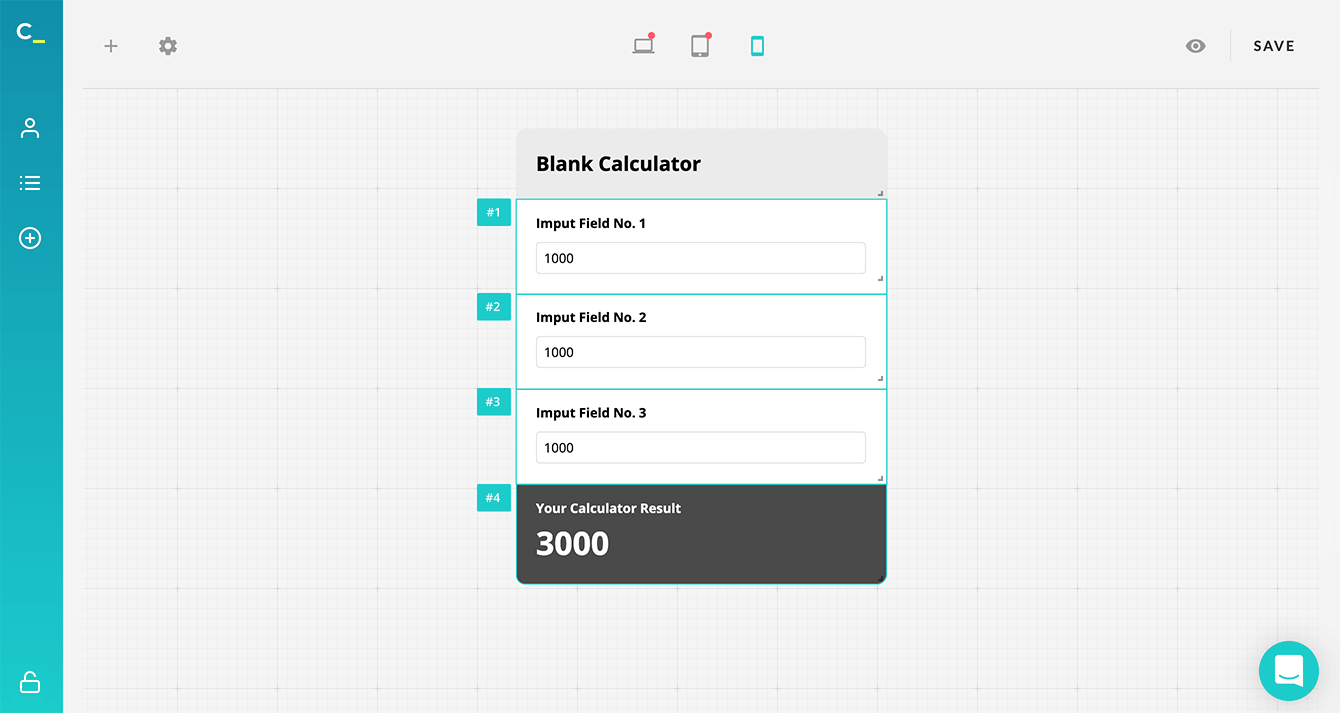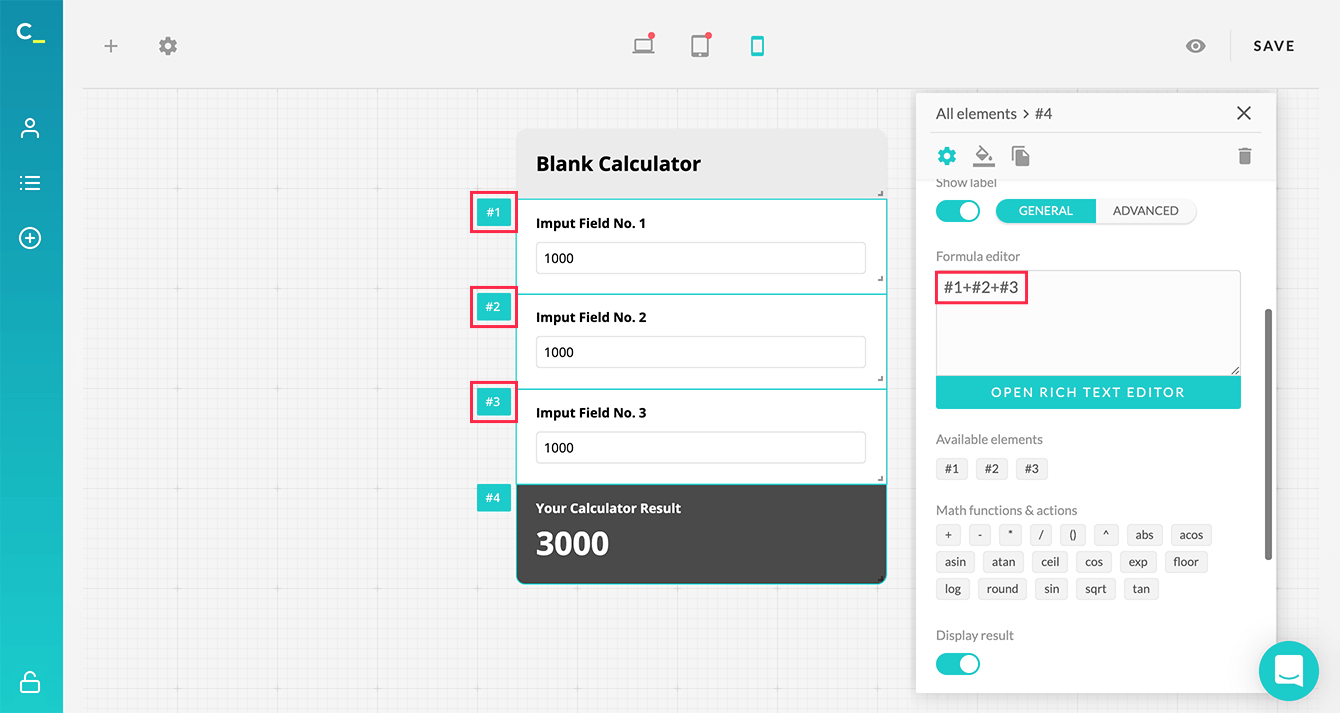keyboard_arrow_left Back to Knowledge Base

# Writing a formula for an online calculator

The formula is probably the most important part of any calculator, and if you know the mathematical formula your calculator is based on (it might also be an Excel formula or something similar), it won't be difficult to convert it for use with Calconic. This article provides an overview of how to understand and write a simple formula for Calconic. Let's get started!

Every single input field you add to your calculator gets its own unique ID. This ID consists of a hashtag and a unique number, like #4. These input fields represent your formula's variables. You can find the ID of a given input field just to the left of it:Let's say you want to create a calculator consisting of three numeric input fields and one result output. Basically, you want your calculator to put together values entered in those three input fields.

First of all, you need to add three Numeric Input Fields. Click the + icon and choose Numeric Input Fields. Name and customize this field according to your needs. Then repeat the process two more times to have three input fields in total. These input fields will each get a unique ID – #1, #2, #3. Then click the + icon again and add the Formula field this time.Now you have a calculator consisting of three numeric input fields and one formula field. The only thing that is missing is an equation connecting all these fields. Click on your formula field and add the following line to the formula editor - #1 + #2 + #3.You’re all set! Your first calculator is ready! Don't forget to Save and Activate your calculator before you embed it to your website. You can also check out our step-by-step tutorial on how to build your first custom calculator!